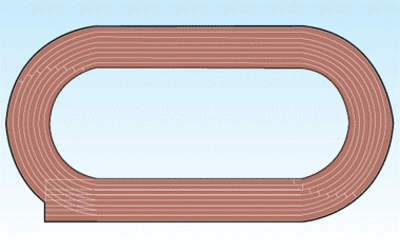# Mrs. Gray's Homework Assignment

Alignments to Content Standards: 5.NF.B.5.b 5.NF.B.4

1/4 mile Track1 lap = 1/4 mile

Part One

Mrs. Gray gave a homework assignment with a fraction problem:

Will ran $1 \frac23$ laps of a $\frac14$ mile track. How far, in miles, did Will run? Jenna and Steve worked together on solving the problem. Jenna said that Will ran about $\frac12$ mile because $1 \frac23 \times \frac14$ is equal to about $\frac12$. Steve answered that Will must have run more than $\frac12$ mile because when you multiply, the product is always larger than the factors and $\frac12$ is not larger than $1 \frac23$.

1. Solve the problem. How far, in miles, did Will run?
2. Is Jenna or Steve correct? Explain your reasoning using words, numbers, and/or pictures.

Part Two

Steve and Jenna continued to work on their homework. The next problems were:

$\frac13 \times 5 =$

$\frac12 \times 2 \frac23 =$

Steve said to Jenna, “Now I get it! When you multiply, the product is always bigger than one of the factors. In the first problem, $\frac13 \times 5$ equals $\frac53$ which is bigger than $\frac13$. In the second problem $\frac12 \times 2 \frac23$ equals $1 \frac13$ which is bigger than $\frac12$."

1. Is Steve’s reasoning correct? Does his rule that the product is always bigger than one of the factors always work?

2. Give at least two examples to prove that Steve is correct or incorrect.

## IM Commentary

This task is intended to assess students’ ability to compare the size of a product to the size of one factor on the basis of the size of the other factor, without performing the indicated multiplication (5.NF.B.5.A). It also assesses students’ ability to explain why multiplying a given number by a fraction greater than 1 results in a product greater than the given number (recognizing multiplication by whole numbers greater than 1 as a familiar case); and explain why multiplying a given number by a fraction less than 1 results in a product smaller than the given number (5.NF.B.5.B). It can be used as an assessment that requires students to multiply fractions (5.NF.B.4). Students will need to have worked with a variety of fraction multiplication situations including whole numbers times fractions, fractions times one, fractions times other fractions less than one, and fractions times other fractions greater than one.

This task was written as part of a collaborative project between Illustrative Mathematics, the Smarter Balanced Digital Library, and the Teaching Channel.

Track image from www.talk-athletics.co.uk

## Solutions

Solution: Part 1

1. $1 \frac23 \times \frac14 = \frac{5}{12}$

Solution: b

1. Jenna is correct because one lap around the track is only $\frac14$ of a mile. When Will ran the track one time he ran $\frac14$ of a mile. When Will ran another $\frac23$ of the track, he ran a little less than $\frac14$ of a mile. Since running the track twice would result in $\frac12$ of a mile, running it a little less than $2$ times would result in a little less than $\frac12$ of a mile, and $\frac{5}{12}$ is a little bit less than $\frac12$ of a mile.

Solution: c

1. Steve’s rule does not always work.

Solution: d

1. When you multiply a fraction less than one times a fraction less than one, the product is always smaller than the two factors. For example: $\frac34 \times \frac14 = \frac{3}{16}$ and $\frac34 \times \frac34 = \frac9{16}$.